Documentation

This is machine translation

Mouseover text to see original. Click the button below to return to the English version of the page.

Compare Surrogate Optimization with Other Solvers

This example compares `surrogateopt` to two other solvers: `fmincon`, the recommended solver for smooth problems, and `patternsearch`, the recommended solver for nonsmooth problems. The example uses a nonsmooth function on a two-dimensional region.

`type nonSmoothFcn`
```function [f, g] = nonSmoothFcn(x) %NONSMOOTHFCN is a non-smooth objective function % Copyright 2005 The MathWorks, Inc. for i = 1:size(x,1) if x(i,1) < -7 f(i) = (x(i,1))^2 + (x(i,2))^2 ; elseif x(i,1) < -3 f(i) = -2*sin(x(i,1)) - (x(i,1)*x(i,2)^2)/10 + 15 ; elseif x(i,1) < 0 f(i) = 0.5*x(i,1)^2 + 20 + abs(x(i,2))+ patho(x(i,:)); elseif x(i,1) >= 0 f(i) = .3*sqrt(x(i,1)) + 25 +abs(x(i,2)) + patho(x(i,:)); end end %Calculate gradient g = NaN; if x(i,1) < -7 g = 2*[x(i,1); x(i,2)]; elseif x(i,1) < -3 g = [-2*cos(x(i,1))-(x(i,2)^2)/10; -x(i,1)*x(i,2)/5]; elseif x(i,1) < 0 [fp,gp] = patho(x(i,:)); if x(i,2) > 0 g = [x(i,1)+gp(1); 1+gp(2)]; elseif x(i,2) < 0 g = [x(i,1)+gp(1); -1+gp(2)]; end elseif x(i,1) >0 [fp,gp] = patho(x(i,:)); if x(i,2) > 0 g = [.15/sqrt(x(i,1))+gp(1); 1+ gp(2)]; elseif x(i,2) < 0 g = [.15/sqrt(x(i,1))+gp(1); -1+ gp(2)]; end end function [f,g] = patho(x) Max = 500; f = zeros(size(x,1),1); g = zeros(size(x)); for k = 1:Max %k arg = sin(pi*k^2*x)/(pi*k^2); f = f + sum(arg,2); g = g + cos(pi*k^2*x); end ```
```mplier = 0.1; % Scale the control variable Objfcn = @(x)nonSmoothFcn(mplier*x); % Handle to the objective function range = [-6 6;-6 6]/mplier; % Range used to plot the objective function rng default % Reset the global random number generator showNonSmoothFcn(Objfcn,range); title('Nonsmooth Objective Function') view(-151,44)```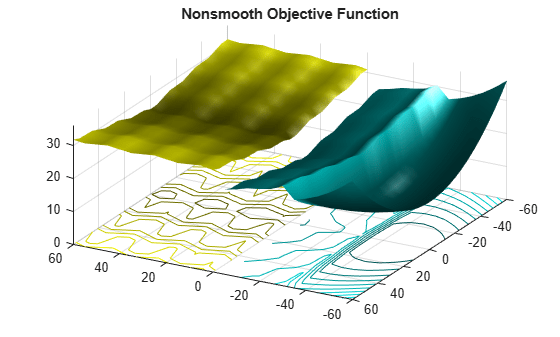`drawnow`

See how well `surrogateopt` does in locating the global minimum within the default number of iterations.

```lb = -6*ones(1,2)/mplier; ub = -lb; [xs,fvals,eflags,outputs] = surrogateopt(Objfcn,lb,ub);``````Surrogateopt stopped because it exceeded the function evaluation limit set by 'options.MaxFunctionEvaluations'. ```
`fprintf("Lowest found value = %g.\r",fvals)`
```Lowest found value = 13. ```
```figure showNonSmoothFcn(Objfcn,range); view(-151,44) hold on p1 = plot3(xs(1),xs(2),fvals,'om','MarkerSize',15,'MarkerFaceColor','m'); legend(p1,{'Solution'}) hold off```Compare with `patternsearch`

Set `patternsearch` options to use the same number of function evaluations, starting from a random point within the bounds.

```rng default x0 = lb + rand(size(lb)).*(ub - lb); optsps = optimoptions('patternsearch','MaxFunctionEvaluations',200,'PlotFcn','psplotbestf'); [xps,fvalps,eflagps,outputps] = patternsearch(Objfcn,x0,[],[],[],[],lb,ub,[],optsps);```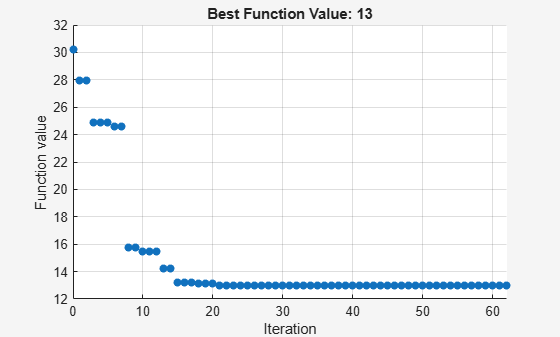```Optimization terminated: mesh size less than options.MeshTolerance. ```
```figure showNonSmoothFcn(Objfcn,range); view(-151,44) hold on p1 = plot3(x0(1),x0(2),Objfcn(x0),'ob','MarkerSize',12,'MarkerFaceColor','b'); p2 = plot3(xps(1),xps(2),fvalps,'om','MarkerSize',15,'MarkerFaceColor','m'); legend([p1,p2],{'Start Point','Solution'}) hold off```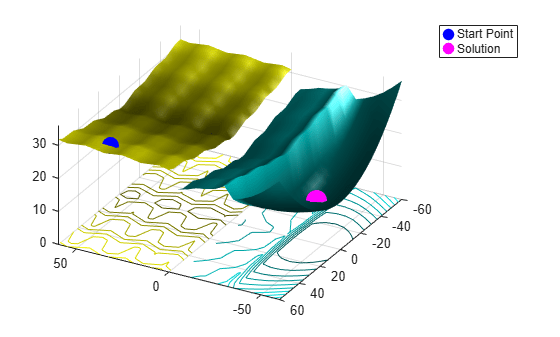`patternsearch` found the same solution as `surrogateopt`.

Restrict the number of function evaluations and try again.

```optsurr = optimoptions('surrogateopt','MaxFunctionEvaluations',40); [xs,fvals,eflags,outputs] = surrogateopt(Objfcn,lb,ub,optsurr);```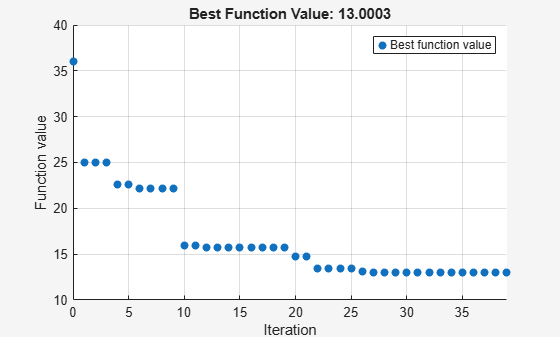```Surrogateopt stopped because it exceeded the function evaluation limit set by 'options.MaxFunctionEvaluations'. ```
```optsps.MaxFunctionEvaluations = 40; [xps,fvalps,eflagps,outputps] = patternsearch(Objfcn,x0,[],[],[],[],lb,ub,[],optsps);```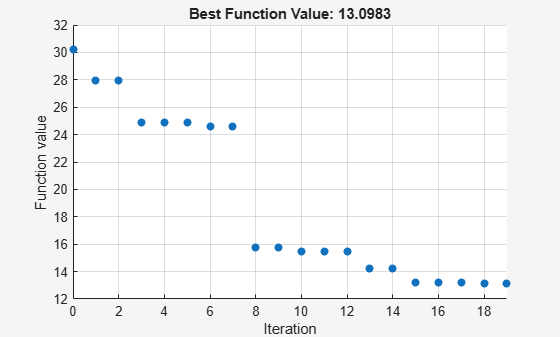```Maximum number of function evaluations exceeded: increase options.MaxFunctionEvaluations. ```

Again, both solvers found the global solution quickly.

Compare with `fmincon`

`fmincon` is efficient at finding a local solution near the start point. However, it can easily get stuck far from the global solution in a nonconvex or nonsmooth problem.

Set `fmincon` options to use a plot function, the same number of function evaluations as the previous solvers, and the same start point as `patternsearch`.

```opts = optimoptions('fmincon','PlotFcn','optimplotfval','MaxFunctionEvaluations',200); [fmsol,fmfval,eflag,fmoutput] = fmincon(Objfcn,x0,[],[],[],[],lb,ub,[],opts);```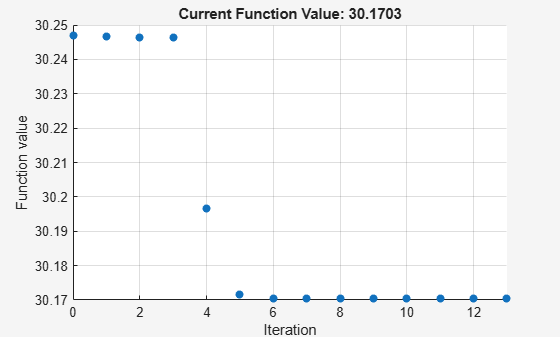```Local minimum possible. Constraints satisfied. fmincon stopped because the size of the current step is less than the value of the step size tolerance and constraints are satisfied to within the value of the constraint tolerance. ```
```figure showNonSmoothFcn(Objfcn,range); view(-151,44) hold on p1 = plot3(x0(1),x0(2),Objfcn(x0),'ob','MarkerSize',12,'MarkerFaceColor','b'); p2 = plot3(fmsol(1),fmsol(2),fmfval,'om','MarkerSize',15,'MarkerFaceColor','m'); legend([p1,p2],{'Start Point','Solution'}) hold off```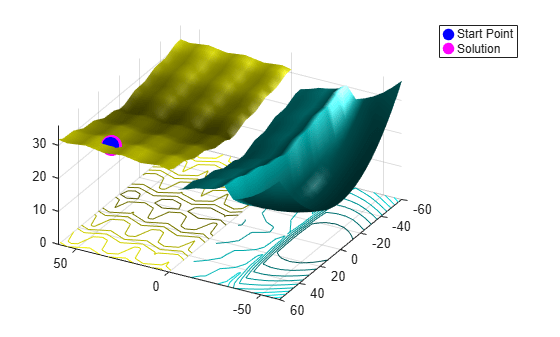`fmincon` is stuck in a local minimum near the start point.